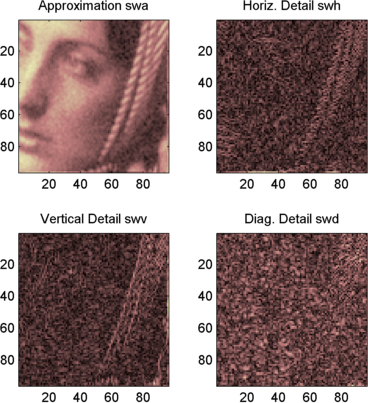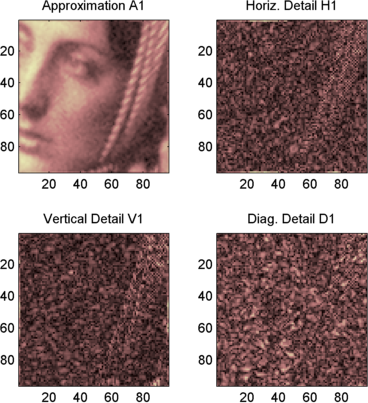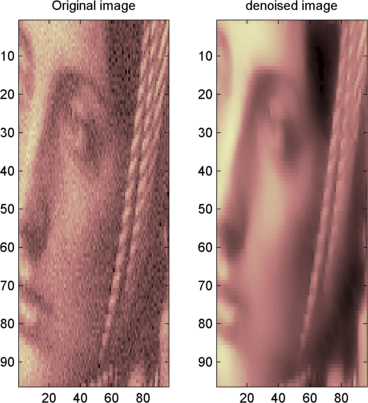## 2-D Stationary Wavelet Transform

This section takes you through the features of 2-D discrete stationary wavelet analysis using the Wavelet Toolbox™ software.

### Analysis-Decomposition Function

Function NamePurpose

`swt2`

Decomposition

### Synthesis-Reconstruction Function

Function NamePurpose

`iswt2`

Reconstruction

The stationary wavelet decomposition structure is more tractable than the wavelet one. So, the utilities useful for the wavelet case are not necessary for the Stationary Wavelet Transform (SWT).

In this section, you'll learn to

• Analyze an image

• Perform single-level and multilevel image decompositions and reconstructions (command line only)

• denoise an image

### 2-D Analysis Using the Command Line

In this example, we'll show how you can use 2-D stationary wavelet analysis to denoise an image.

Note

Instead of using `image(I)` to visualize the image `I`, we use` image(wcodemat(I))`, which displays a rescaled version of `I` leading to a clearer presentation of the details and approximations (see the `wcodemat` reference page).

This example involves a image containing noise.

From the MATLAB® prompt, type

```load noiswom whos ```
NameSizeBytesClass
`X``96x96``73728``double array`
`map``255x3``6120``double array`

For the SWT, if a decomposition at level `k` is needed, `2^k` must divide evenly into `size(X,1)` and `size(X,2)`. If your original image is not of correct size, you can use the function `wextend` to extend it.

2. Perform a single-level Stationary Wavelet Decomposition.

Perform a single-level decomposition of the image using the `db1` wavelet. Type

```[swa,swh,swv,swd] = swt2(X,1,'db1'); ```

This generates the coefficients matrices of the level-one approximation (`swa`) and horizontal, vertical and diagonal details (`swh`, `swv`, and `swd`, respectively). Both are of size-the-image size. Type

```whos ```
NameSizeBytesClass
`X``96x96``73728``double array`
`map``255x3``6120``double array`
`swa``96x96``73728``double array`
`swh``96x96``73728``double array`
`swv``96x96``73728``double array`
`swd``96x96``73728``double array`
3. Display the coefficients of approximation and details.

To display the coefficients of approximation and details at level 1, type

```map = pink(size(map,1)); colormap(map) subplot(2,2,1), image(wcodemat(swa,192)); title('Approximation swa') subplot(2,2,2), image(wcodemat(swh,192)); title('Horiz. Detail swh') subplot(2,2,3), image(wcodemat(swv,192)); title('Vertical Detail swv') subplot(2,2,4), image(wcodemat(swd,192)); title('Diag. Detail swd'); ```4. Regenerate the image by Inverse Stationary Wavelet Transform.

To find the inverse transform, type

```A0 = iswt2(swa,swh,swv,swd,'db1'); ```

To check the perfect reconstruction, type

```err = max(max(abs(X-A0))) err = 1.1369e-13 ```
5. Construct and display approximation and details from the coefficients.

To construct the level 1 approximation and details (`A1`, `H1`, `V1` and `D1`) from the coefficients `swa`, `swh`, `swv` and `swd`, type

```nulcfs = zeros(size(swa)); A1 = iswt2(swa,nulcfs,nulcfs,nulcfs,'db1'); H1 = iswt2(nulcfs,swh,nulcfs,nulcfs,'db1'); V1 = iswt2(nulcfs,nulcfs,swv,nulcfs,'db1'); D1 = iswt2(nulcfs,nulcfs,nulcfs,swd,'db1'); ```

To display the approximation and details at level 1, type

```colormap(map) subplot(2,2,1), image(wcodemat(A1,192)); title('Approximation A1') subplot(2,2,2), image(wcodemat(H1,192)); title('Horiz. Detail H1') subplot(2,2,3), image(wcodemat(V1,192)); title('Vertical Detail V1') subplot(2,2,4), image(wcodemat(D1,192)); title('Diag. Detail D1') ```6. Perform a multilevel Stationary Wavelet Decomposition.

To perform a decomposition at level 3 of the image (again using the `db1` wavelet), type

```[swa,swh,swv,swd] = swt2(X,3,'db1'); ```

This generates the coefficients of the approximations at levels 1, 2, and 3 (`swa`) and the coefficients of the details (`swh`, `swv` and `swd`). Observe that the matrices `swa(:,:,i)`, `swh(:,:,i)`, `swv(:,:,i)`, and `swd(:,:,i)` for a given level `i` are of size-the-image size. Type

```clear A0 A1 D1 H1 V1 err nulcfs whos ```
NameSizeBytesClass
`X``96x96``73728``double array`
`map``255x3``6120``double array`
`swa``96x96x3``221184``double array`
`swh``96x96x3``221184``double array`
`swv``96x96x3``221184``double array`
`swd``96x96x3``221184``double array`
7. Display the coefficients of approximations and details.

To display the coefficients of approximations and details, type

```colormap(map) kp = 0; for i = 1:3 subplot(3,4,kp+1), image(wcodemat(swa(:,:,i),192)); title(['Approx. cfs level ',num2str(i)]) subplot(3,4,kp+2), image(wcodemat(swh(:,:,i),192)); title(['Horiz. Det. cfs level ',num2str(i)]) subplot(3,4,kp+3), image(wcodemat(swv(:,:,i),192)); title(['Vert. Det. cfs level ',num2str(i)]) subplot(3,4,kp+4), image(wcodemat(swd(:,:,i),192)); title(['Diag. Det. cfs level ',num2str(i)]) kp = kp + 4; end ```
8. Reconstruct approximation at Level 3 and details from coefficients.

To reconstruct the approximation at level 3, type

```mzero = zeros(size(swd)); A = mzero; A(:,:,3) = iswt2(swa,mzero,mzero,mzero,'db1'); ```

To reconstruct the details at levels 1, 2 and 3, type

```H = mzero; V = mzero; D = mzero; for i = 1:3 swcfs = mzero; swcfs(:,:,i) = swh(:,:,i); H(:,:,i) = iswt2(mzero,swcfs,mzero,mzero,'db1'); swcfs = mzero; swcfs(:,:,i) = swv(:,:,i); V(:,:,i) = iswt2(mzero,mzero,swcfs,mzero,'db1'); swcfs = mzero; swcfs(:,:,i) = swd(:,:,i); D(:,:,i) = iswt2(mzero,mzero,mzero,swcfs,'db1'); end ```
9. Reconstruct and display approximations at Levels 1, 2 from approximation at Level 3 and details at Levels 1, 2, and 3.

To reconstruct the approximations at levels 2 and 3, type

```A(:,:,2) = A(:,:,3) + H(:,:,3) + V(:,:,3) + D(:,:,3); A(:,:,1) = A(:,:,2) + H(:,:,2) + V(:,:,2) + D(:,:,2); ```

To display the approximations and details at levels 1, 2, and 3, type

```colormap(map) kp = 0; for i = 1:3 subplot(3,4,kp+1), image(wcodemat(A(:,:,i),192)); title(['Approx. level ',num2str(i)]) subplot(3,4,kp+2), image(wcodemat(H(:,:,i),192)); title(['Horiz. Det. level ',num2str(i)]) subplot(3,4,kp+3), image(wcodemat(V(:,:,i),192)); title(['Vert. Det. level ',num2str(i)]) subplot(3,4,kp+4), image(wcodemat(D(:,:,i),192)); title(['Diag. Det. level ',num2str(i)]) kp = kp + 4; end ```
10. Remove noise by thresholding.

To denoise an image, use the threshold value we find using the Wavelet Analyzer app tool (see the next section), use the `wthresh` command to perform the actual thresholding of the detail coefficients, and then use the `iswt2` command to obtain the denoised image.

```thr = 44.5; sorh = 's'; dswh = wthresh(swh,sorh,thr); dswv = wthresh(swv,sorh,thr); dswd = wthresh(swd,sorh,thr); clean = iswt2(swa,dswh,dswv,dswd,'db1'); ```

To display both the original and denoised images, type

```colormap(map) subplot(1,2,1), image(wcodemat(X,192)); title('Original image') subplot(1,2,2), image(wcodemat(clean,192)); title('denoised image') ```A second syntax can be used for the `swt2` and `iswt2` functions, giving the same results:

```lev= 4; swc = swt2(X,lev,'db1'); swcden = swc; swcden(:,:,1:end-1) = wthresh(swcden(:,:,1:end-1),sorh,thr); clean = iswt2(swcden,'db1'); ```

You obtain the same plot by using the plot commands in step 9 above.

### Interactive 2-D Stationary Wavelet Transform Denoising

In this section, we explore a strategy for denoising images based on the 2-D stationary wavelet analysis using the Wavelet Analyzer app. The basic idea is to average many slightly different discrete wavelet analyses.

1. Start the Stationary Wavelet Transform Denoising 2-D Tool.

From the MATLAB prompt, type `waveletAnalyzer`.

The Wavelet Analyzer appears:Click the SWT Denoising 2-D menu item.At the MATLAB command prompt, type

`load noiswom`
In the SWT Denoising 2-D tool, select File > Import Image from Workspace. When the Import from Workspace dialog box appears, select the `X` variable. Click OK to import the image.

3. Perform a Stationary Wavelet Decomposition.

Select the `haar` wavelet from the Wavelet menu, select 4 from the Level menu, and then click the Decompose Image button.

The tool displays the histograms of the stationary wavelet detail coefficients of the image on the left of the window. These histograms are organized as follows:

• From the bottom for level 1 to the top for level 4

• On the left horizontal coefficients, in the middle diagonal coefficients, and on the right vertical coefficients

4. Denoise the image using the Stationary Wavelet Transform.

While a number of options are available for fine-tuning the denoising algorithm, we'll accept the defaults of fixed form soft thresholding and unscaled white noise. The sliders located to the right of the window control the level dependent thresholds indicated by the dashed lines running vertically through the histograms of the coefficients on the left of the window. Click the Denoise button.The result seems to be oversmoothed and the selected thresholds too aggressive. Nevertheless, the histogram of the residuals is quite good since it is close to a Gaussian distribution, which is the noise introduced to produce the analyzed image `noiswom.mat` from a piece of the original image `woman.mat`.

5. Selecting a thresholding method.

From the Select thresholding method menu, choose the Penalize low item. The associated default for the thresholding mode is automatically set to hard; accept it. Use the Sparsity slider to adjust the threshold value close to 45.5, and then click the denoise button.The result is quite satisfactory, although it is possible to improve it slightly.

Select the `sym6` wavelet and click the Decompose Image button. Use the Sparsity slider to adjust the threshold value close to 40.44, and then click the denoise button.

### Importing and Exporting Information from the Wavelet Analyzer App

The tool lets you save the denoised image to disk. The toolbox creates a MAT-file in the current folder with a name you choose.

To save the denoised image from the present denoising process, use the menu File > Save denoised Image. A dialog box appears that lets you specify a folder and filename for storing the image. Type the name `dnoiswom`. After saving the image data to the file `dnoiswom.mat`, load the variables into your workspace:

```load dnoiswom whos ```
NameSizeBytesClass
`X``96x96``73728``double array`
`map``255x3``6120``double array`
`valTHR``3x4``96``double array`
`wname``1x4``8``char array`

The denoised image is `X` and `map` is the colormap. In addition, the parameters of the denoising process are available. The wavelet name is contained in `wname`, and the level dependent thresholds are encoded in `valTHR`. The variable `valTHR` has four columns (the level of the decomposition) and three rows (one for each detail orientation).